# Lab 5.1 IF Statements

 Team-Fly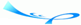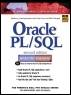Oracle® PL/SQL® Interactive Workbook, Second EditionBy Benjamin Rosenzweig, Elena Silvestrova Table of Contents Chapter 5.  Conditional Control: IF Statements

Lab Objectives

After this Lab, you will be able to:

• Use IF-THEN Statements

• Use IF-THEN-ELSE Statements"/>

An IF statement has two forms: IF-THEN and IF-THEN-ELSE. An IF-THEN statement allows you to specify only one group of actions to take. In other words, this group of actions is taken only when a condition evaluates to TRUE. An IF-THEN-ELSE statement allows you to specify two groups of actions, and the second group of actions is taken when a condition evaluates to FALSE or NULL.

#### IF-THEN Statements

An IF-THEN statement is the most basic kind of a conditional control and has the following structure:

` IF CONDITION THEN     STATEMENT 1;     …     STATEMENT N;  END IF; `

The reserved word IF marks the beginning of the IF statement. Statements 1 through N are a sequence of executable statements that consist of one or more of the standard programming structures. The word CONDITION between keywords IF and THEN determines whether these statements are executed. END IF is a reserved phrase that indicates the end of the IF-THEN construct.

This flow of the logic from the preceding structure of the IF-THEN statement is illustrated in the Figure 5.1.

##### Figure 5.1. IF-THEN Statement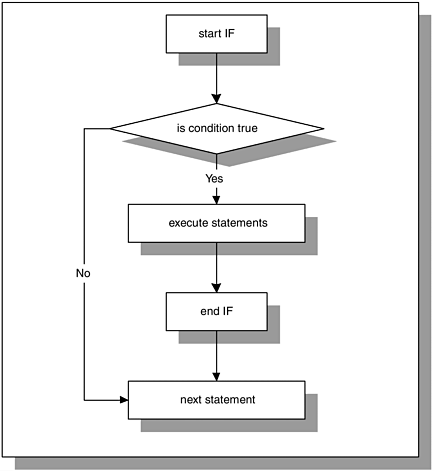When an IF-THEN statement is executed, a condition is evaluated to either TRUE or FALSE. If the condition evaluates to TRUE, control is passed to the first executable statement of the IF-THEN construct. If the condition evaluates to FALSE, control is passed to the first executable statement after the END IF statement.

Consider the following example. You have two numeric values stored in the variables, v_num1 and v_num2. You need to arrange your values so that the smaller value is always stored in v_num1, and the larger value is always stored in the v_num2.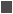FOR EXAMPLE

` DECLARE     v_num1 NUMBER := 5;     v_num2 NUMBER := 3;     v_temp NUMBER;  BEGIN     -- if v_num1 is greater than v_num2 rearrange their values     IF v_num1 > v_num2 THEN        v_temp := v_num1;        v_num1 := v_num2;        v_num2 := v_temp;     END IF;     -- display the values of v_num1 and v_num2     DBMS_OUTPUT.PUT_LINE ('v_num1 = '||v_num1);     DBMS_OUTPUT.PUT_LINE ('v_num2 = '||v_num2);  END; `

In this example, condition v_num1 > v_num2 evaluates to TRUE because 5 is greater that 3. Next, the values are rearranged so that 3 is assigned to v_num1, and 5 is assigned to v_num2. It is done with the help of the third variable, v_temp, which is used for temporary storage.

This example produces the following output:

` v_num1 = 3  v_num2 = 5  PL/SQL procedure successfully completed. `

#### IF-THEN-ELSE Statement

An IF-THEN statement specifies the sequence of statements to execute only if the condition evaluates to TRUE. When this condition evaluates to FALSE, there is no special action to take except to proceed with execution of the program.

An IF-THEN-ELSE statement enables you to specify two groups of statements. One group of statements is executed when the condition evaluates to TRUE. Another group of statements is executed when the condition evaluates to FALSE. This is indicated as follows:

` IF CONDITION THEN     STATEMENT 1;  ELSE     STATEMENT 2;  END IF;  STATEMENT 3; `

When CONDITION evaluates to TRUE, control is passed to STATEMENT 1; when CONDITION evaluates to FALSE, control is passed to STATEMENT 2. After the IF-THEN-ELSE construct has completed, STATEMENT 3 is executed. This flow of the logic is illustrated in the Figure 5.2.

##### Figure 5.2. IF-THEN-ELSE Statement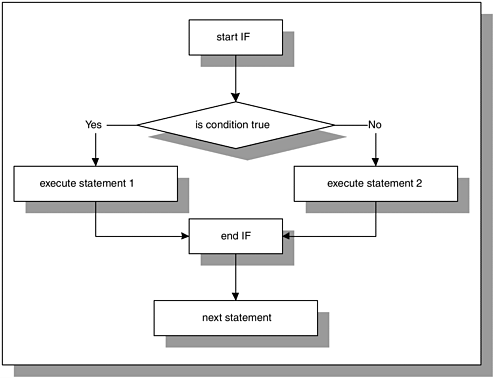The IF-THEN-ELSE construct should be used when trying to choose between two mutually exclusive actions. Consider the following example:

` DECLARE     v_num NUMBER := &sv_user_num;  BEGIN     -- test if the number provided by the user is even     IF MOD(v_num,2) = 0  THEN        DBMS_OUTPUT.PUT_LINE (v_num||' is even number');     ELSE        DBMS_OUTPUT.PUT_LINE (v_num||' is odd number');     END IF;     DBMS_OUTPUT.PUT_LINE ('Done');  END; `

It is important to realize that for any given number only one of the DBMS_ OUTPUT.PUT_LINE statements is executed. Hence, the IF-THEN-ELSE construct enables you to specify two and only two mutually exclusive actions.

When run, this example produces the following output:

` Enter value for v_user_num: 24  old   2:    v_num  NUMBER := &v_user_num;  new   2:    v_num  NUMBER := 24;  24 is even number  Done  PL/SQL procedure successfully completed. `

#### NULL Condition

In some cases, a condition used in an IF statement can be evaluated to NULL instead of TRUE or FALSE. For the IF-THEN construct, the statements will not be executed if an associated condition evaluates to NULL. Next, control will be passed to the first executable statement after END IF. For the IF-THEN-ELSE construct, the statements specified after the keyword ELSE will be executed if an associated condition evaluates to NULL.FOR EXAMPLE

` DECLARE     v_num1 NUMBER := 0;     v_num2 NUMBER;  BEGIN     IF v_num1 = v_num2 THEN        DBMS_OUTPUT.PUT_LINE ('v_num1 = v_num2');     ELSE        DBMS_OUTPUT.PUT_LINE ('v_num1 != v_num2');     END IF;  END; `

This example produces the following output:

` v_num1 != v_num2  PL/SQL procedure successfully completed. `

The condition

` v_num1 = v_num2 `

is evaluated to NULL because a value is not assigned to the variable v_num2. Therefore, variable v_num2 is NULL. Notice that the IF-THEN-ELSE construct is behaving as if the condition evaluated to FALSE, and the second DBMS_ OUTPUT.PUT_LINE statement is executed.

#### Lab 5.1 Exercises

##### 5.1.1 Use the IF-THEN Statement

In this exercise, you will use the IF-THEN statement to test whether the date provided by the user falls on the weekend. In other words, if the day happens to be Saturday or Sunday.

Create the following PL/SQL script:

` -- ch05_1a.sql, version 1.0  SET SERVEROUTPUT ON  DECLARE     v_date DATE := TO_DATE('&sv_user_date', 'DD-MON-YYYY');     v_day VARCHAR2(15);  BEGIN     v_day := RTRIM(TO_CHAR(v_date, 'DAY'));     IF v_day IN ('SATURDAY', 'SUNDAY') THEN        DBMS_OUTPUT.PUT_LINE (v_date||' falls on weekend');     END IF;     --- control resumes here     DBMS_OUTPUT.PUT_LINE ('Done…');  END; `

In order to test this script fully, execute it twice. For the first run, enter '09-JAN-2002', and for the second run, enter '13-JAN-2002'. Execute the script, and then answer the following questions:

 a) What output was printed on the screen (for both dates)? b) Explain why the output produced for the two dates is different.

Remove the RTRIM function from the assignment statement for v_day as follows:

` v_day := TO_CHAR(v_date, 'DAY'); `

Run the script again, entering '13-JAN-2002' for v_date.

 c) What output was printed on the screen? Why? d) Rewrite this script using the LIKE operator instead of the IN operator, so that it produces the same results for the dates specified earlier. e) Rewrite this script using the IF-THEN-ELSE construct. If the date specified does not fall on the weekend, display a message to the user saying so.

##### 5.1.2 Use the IF-THEN-ELSE Statement

In this exercise, you will use the IF-THEN-ELSE statement to check how many students are enrolled in course number 25, section 1. If there are 15 or more students enrolled, section 1 of course number 25 is full. Otherwise, section 1 of course number 25 is not full and more students can register for it. In both cases, a message should be displayed to the user indicating whether section 1 is full. Try to answer the questions before you run the script. Once you have answered the questions, run the script and check your answers. Note that the SELECT INTO statement uses ANSI 1999 SQL standard.

Create the following PL/SQL script:

` -- ch05_2a.sql, version 1.0  SET SERVEROUTPUT ON  DECLARE     v_total NUMBER;  BEGIN     SELECT COUNT(*)       INTO v_total       FROM enrollment e       JOIN section s USING (section_id)      WHERE s.course_no = 25        AND s.section_no = 1;     -- check if section 1 of course 25 is full     IF v_total >= 15 THEN        DBMS_OUTPUT.PUT_LINE           ('Section 1 of course 25 is full');     ELSE        DBMS_OUTPUT.PUT_LINE           ('Section 1 of course 25 is not full');     END IF;     -- control resumes here  END; `

Notice that the SELECT INTO statement uses an equijoin. The join condition is listed in the JOIN clause, indicating columns that are part of the primary key and foreign key constraints. In this example, column SECTION_ID of the ENROLLMENT table has a foreign key constraint defined on it. This constraint references column SECTION_ID of the SECTION table, which, in turn, has a primary key constraint defined on it.You will find detailed explanations and examples of the statements using new ANSI 1999 SQL standard in Appendix E and in the Oracle help. Throughout this book we try to provide you with examples illustrating both standards; however, our main focus is on PL/SQL features rather than SQL.

In the previous versions of Oracle, this statement would look as follows:

` SELECT COUNT(*)    INTO v_total    FROM enrollment e, section s   WHERE e.section_id = s.section_id     AND s.course_no = 25     AND s.section_no = 1; `

Try to answer the following questions first and then execute the script:

 a) What DBMS_OUTPUT.PUT_LINE statement will be displayed if there are 15 students enrolled in section 1 of course number 25? b) What DBMS_OUTPUT.PUT_LINE statement will be displayed if there are 3 students enrolled in section 1 of course number 25? c) What DBMS_OUTPUT.PUT_LINE statement will be displayed if there is no section 1 for course number 25? d) How would you change this script so that both course and section numbers are provided by a user? e) How would you change this script so that if there are less than 15 students enrolled in section 1 of course number 25, a message indicating how many students can still be enrolled is displayed?

#### Lab 5.1 Exercise Answers

This section gives you some suggested answers to the questions in Lab 5.1, with discussion related to how those answers resulted. The most important thing to realize is whether your answer works. You should figure out the implications of the answers here and what the effects are from any different answers you may come up with.

##### 5.1.1 Answers

 a) What output was printed on the screen (for both dates)? A1: Answer: The first output produced for the date is 09-JAN-2002. The second output produced for the date is 13-JAN-2002.` Enter value for sv_user_date: 09-JAN-2002 old 2: v_date DATE := TO_DATE('&sv_user_date', 'DD-MON- YYYY'); new 2: v_date DATE := TO_DATE('09-JAN-2002', 'DD-MON- YYYY'); Done... PL/SQL procedure successfully completed. `When the value of 09-JAN-2002 is entered for v_date, the day of the week is determined for the variable v_day with the help of the functions TO_CHAR and RTRIM. Next, the following condition is evaluated: ` v_day IN ('SATURDAY', 'SUNDAY') `Because the value of v_day is 'WEDNESDAY,' the condition evaluates to FALSE. Then, control is passed to the first executable statement after END IF. As a result, 'Done…' is displayed on the screen. ` Enter value for sv_user_date: 13-JAN-2002 old 2: v_date DATE := TO_DATE('&sv_user_date', 'DD-MON- YYYY'); new 2: v_date DATE := TO_DATE('13-JAN-2002', 'DD-MON- YYYY'); 13-JAN-02 falls on weekend Done... PL/SQL procedure successfully completed. `The value of v_day is derived from the value of v_date. Next, the condition of the IF-THEN statement is evaluated. Because it evaluates to TRUE, the statement after the keyword THEN is executed. So, '13-JAN-2002 falls on weekend' is displayed on the screen. Next, control is passed to the last DBMS_OUTPUT.PUT_LINE statement, and 'Done . . .' is displayed on the screen. b) Explain why the output produced for the two dates is different. A2: Answer: The first date, 09-JAN-2002, is a Wednesday. As a result, the condition, v_day IN ('SATURDAY,' 'SUNDAY'), does not evaluate to TRUE. So, control is transferred to the statement after END IF, and 'Done...' is displayed on the screen.The second date, 13-JAN-2002, is a Sunday. Because Sunday falls on a weekend, the condition evaluates to TRUE, and the message '13-JAN-2002 falls on weekend' is displayed on the screen. Next, the last DBMS_OUTPUT.PUT_LINE statement is executed, and 'Done...'is displayed on the screen.Remove the RTRIM function from the assignment statement for v_day as follows: ` v_day := TO_CHAR(v_date, 'DAY'); `Run the script again, entering '13-JAN-2002' for v_date.

 c) What output was printed on the screen? Why? A1: Answer: Your script should look similar to the following. Changes are shown in bold letters.` -- ch05_1b.sql, version 2.0 SET SERVEROUTPUT ON DECLARE v_date DATE := TO_DATE('&sv_user_date', 'DD-MON-YYYY'); v_day VARCHAR2(15); BEGIN v_day := TO_CHAR(v_date, 'DAY'); IF v_day IN ('SATURDAY', 'SUNDAY') THEN DBMS_OUTPUT.PUT_LINE (v_date||' falls on weekend'); END IF; --- control resumes here DBMS_OUTPUT.PUT_LINE ('Done…'); END; `This script produces the following output: ` Enter value for sv_user_date: 13-JAN-2002 old 2: v_date DATE := TO_DATE('&sv_user_date', 'DD-MON- YYYY'); new 2: v_date DATE := TO_DATE('13-JAN-2002', 'DD-MON- YYYY'); Done... PL/SQL procedure successfully completed. `In the original example, the variable v_day is calculated with the help of the statement, RTRIM(TO_CHAR(v_date, 'DAY')). First, the function TO_CHAR returns the day of the week padded with blanks. The size of the value retrieved by the function TO_CHAR is always 9 bytes. Next, the RTRIM function removes trailing spaces.In the statement ` v_day := TO_CHAR(v_date, 'DAY') `the TO_CHAR function is used without the RTRIM function. Therefore, trailing blanks are not removed after the day of the week has been derived. As a result, the condition of the IF-THEN statement evaluates to FALSE even though given date falls on the weekend, and control is passed to the last DBMS_ OUTPUT.PUT_LINE statement. d) Rewrite this script using the LIKE operator instead of the IN operator, so that it produces the same results for the dates specified earlier. A2: Answer: Your script should look similar to the following. Changes are shown in bold letters.` -- ch05_1c.sql, version 3.0 SET SERVEROUTPUT ON DECLARE v_date DATE := TO_DATE('&sv_user_date', 'DD-MON-YYYY'); v_day VARCHAR2(15); BEGIN v_day := RTRIM(TO_CHAR(v_date, 'DAY')); IF v_day LIKE 'S%' THEN DBMS_OUTPUT.PUT_LINE (v_date||' falls on weekend'); END IF; --- control resumes here DBMS_OUTPUT.PUT_LINE ('Done…'); END; `Both days, Saturday and Sunday, are the only days of the week that start with the letter 'S'. As a result, there is no need to spell out the names of the days or specify any additional letters for the LIKE operator. e) Rewrite this script using the IF-THEN-ELSE construct. If the date specified does not fall on the weekend, display a message to the user saying so. A3: Answer: Your script should look similar to the following. Changes are shown in bold letters. ` -- ch05_1d.sql, version 4.0 SET SERVEROUTPUT ON DECLARE v_date DATE := TO_DATE('&sv_user_date', 'DD-MON-YYYY'); v_day VARCHAR2(15); BEGIN v_day := RTRIM(TO_CHAR(v_date, 'DAY')); IF v_day IN ('SATURDAY', 'SUNDAY') THEN DBMS_OUTPUT.PUT_LINE (v_date||' falls on weekend'); ELSE DBMS_OUTPUT.PUT_LINE (v_date|| ' does not fall on the weekend'); END IF; -- control resumes here DBMS_OUTPUT.PUT_LINE('Done…'); END; `In order to modify the script, the ELSE part was added to the IF statement. The rest of the script has not been changed.

##### 5.1.2 Answers

 a) What DBMS_OUTPUT.PUT_LINE statement will be displayed if there are 15 students enrolled in section 1 of course number 25? A1: Answer: If there are 15 or more students enrolled in section 1 of course number 25, the first DBMS_OUTPUT.PUT_LINE statement is displayed on the screen.The condition ` v_total >= 15 `evaluates to TRUE, and as a result, the statement ` DBMS_OUTPUT.PUT_LINE ('Section 1 of course 25 is full'); `is executed. b) What DBMS_OUTPUT.PUT_LINE statement will be displayed if there are 3 students enrolled in section 1 of course number 25? A2: Answer: If there are 3 students enrolled in section 1 of course number 25, the second DBMS_OUTPUT.PUT_LINE statement is displayed on the screen.The condition ` v_total >= 15 `evaluates to FALSE, and the ELSE part on the IF-THEN-ELSE statement is executed. As a result, the statement ` DBMS_OUTPUT.PUT_LINE ('Section 1 of course 25 is not full'); `is executed. c) What DBMS_OUTPUT.PUT_LINE statement will be displayed if there is no section 1 for course number 25? A3: Answer: If there is no section 1 for course number 25, the ELSE part of the IF-THEN-ELSE statement will be executed. So the second DBMS_OUTPUT.PUT_LINE statement will be displayed on the screen.The COUNT function used in the SELECT statement ` SELECT COUNT(*) INTO v_total FROM enrollment e JOIN section s USING (section_id) WHERE s.course_no = 25 AND s.section_no = 1; `returns 0. The condition of the IF-THEN-ELSE statement evaluates to FALSE. Therefore, the ELSE part of the IF-THEN-ELSE statement is executed, and the second DBMS_OUTPUT.PUT_LINE statement is displayed on the screen. d) How would you change this script so that both course and section numbers are provided by a user? A4: Answer: Two additional variables must be declared and initialized with the help of the substitution variables as follows. Your script should look similar to this script. Changes are shown in bold letters.` -- ch05_2b.sql, version 2.0 SET SERVEROUTPUT ON DECLARE v_total NUMBER; v_course_no CHAR(6) := '&sv_course_no'; v_section_no NUMBER := &sv_section_no; BEGIN SELECT COUNT(*) INTO v_total FROM enrollment e JOIN section s USING (section_id) WHERE s.course_no = v_course_no AND s.section_no = v_section_no; -- check if a specific section of a course is full IF v_total >= 15 THEN DBMS_OUTPUT.PUT_LINE ('Section 1 of course 25 is full'); ELSE DBMS_OUTPUT.PUT_LINE ('Section 1 of course 25 is not full'); END IF; -- control resumes here END; ` e) How would you change this script so that if there are less than 15 students enrolled in section 1 of course number 25, a message indicating how many students can still be enrolled is displayed? A5: Answer: Your script should look similar to this script. Changes are shown in bold letters.` -- ch05_2c.sql, version 3.0 SET SERVEROUTPUT ON DECLARE v_total NUMBER; v_students NUMBER; BEGIN SELECT COUNT(*) INTO v_total FROM enrollment e JOIN section s USING (section_id) WHERE s.course_no = 25 AND s.section_no = 1; -- check if section 1 of course 25 is full IF v_total >= 15 THEN DBMS_OUTPUT.PUT_LINE ('Section 1 of course 25 is full'); ELSE v_students := 15 v_total; DBMS_OUTPUT.PUT_LINE (v_students|| ' students can still enroll into section 1 '|| 'of course 25'); END IF; -- control resumes here END; `Notice that if the IF-THEN-ELSE statement evaluates to FALSE, the statements associated with the ELSE part are executed. In this case, the value of the variable v_total is subtracted from 15. The result of this operation indicates how many more students can enroll in section 1 of course number 25.

#### Lab 5.1 Self-Review Questions

In order to test your progress, you should be able to answer the following questions.

Answers appear in Appendix A, Section 5.1.

 1) An IF construct is a control statement for which of the following? _____ Sequence structure_____ Iteration structure_____ Selection structure 2) In order for the statements of an IF-THEN construct to be executed, the condition must evaluate to which of the following? _____ TRUE_____ FALSE_____ NULL 3) When a condition of the IF-THEN-ELSE construct is evaluated to NULL, control is passed to the first executable statement after END IF. _____ True_____ False 4) How many actions can you specify in an IF-THEN-ELSE statement? _____ One_____ Two_____ Four_____ As many as you require 5) The IF-THEN-ELSE construct should be used to achieve which of the following? _____ Three mutually exclusive actions_____ Two mutually exclusive actions_____ Two actions that are not mutually exclusive

 Team-FlyTop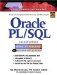Oracle PL/SQL Interactive Workbook (2nd Edition)
ISBN: 0130473200
EAN: 2147483647
Year: 2002
Pages: 146

Similar book on Amazon

flylib.com © 2008-2017.
If you may any questions please contact us: flylib@qtcs.net